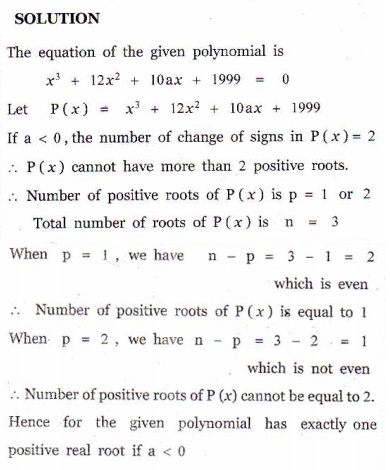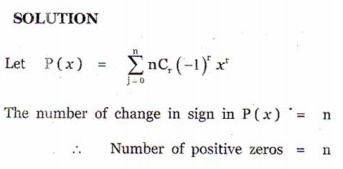Home | | Maths 12th Std | Choose the correct Answers

## Chapter: 12th Mathematics : UNIT 3 : Theory of Equations

Choose the correct or the most suitable answer from the given four alternatives, Multiple choice questions with Answers, Solution - Maths Book back 1 mark questions and answers with solution for Exercise Problems

Choose the correct or the most suitable answer from the given four alternatives :

1. A zero of x+ 64 is

(1) 0

(2) 4

(3) 4i

(4) -42 If and are polynomials of degrees and respectively, and if h(x= ( o )(x) , then the degree of is

(1) mn

(2) m + n

(3) mn

(4) nm3. A polynomial equation in of degree always has

(1) n distinct roots

(2) n real roots

(3) n imaginary roots

(4) at most one root.4. If α β , and  γ  are the zeros of  x3  + px2  + qx + , thenis

(1) -q/r

(2) -p/r

(3) q/r

(4) -q/p5. According to the rational root theorem, which number is not possible rational zero of 4x7  + 2x10x 5 ?

(1) −1

(2) 5/4

(3) 4/5

(4) 56. The polynomial x kx+ 9x has three real zeros if and only if, satisfies

(1) |k| ≤ 6

(2) k = 0

(3) |k| > 6

(4) |k| ≥ 67. The number of real numbers in [0, 2π] satisfying sin 2 sin+1 is

(1) 2

(2) 4

(3) 1

(4) ∞8. If x+12x+10ax +1999 definitely has a positive zero, if and only if

(1) a ≥ 0

(2) a > 0

(3) a < 0

(4) a ≤ 09. The polynomial x+ 2+ 3 has

(1) one negative and two imaginary zeros

(2) one positive and two imaginary zeros

(3) three real zeros

(4) no zeros10. The number of positive zeros of the polynomialis

(1) 0

(2) n

(3) < n

(4) rChoose the correct or the most suitable answer from the given four alternatives :

1. A zero of x+ 64 is

(1) 0

(2) 4

(3) 4i

(4) -4

2 If and are polynomials of degrees and respectively, and if h(x= ( o )(x) , then the degree of is

(1) mn

(2) m + n

(3) mn

(4) nm

3. A polynomial equation in of degree always has

(1) n distinct roots

(2) n real roots

(3) n imaginary roots

(4) at most one root.

4. If α β , and  γ  are the zeros of  x3  + px2  + qx + , thenis

(1) -q/r

(2) -p/r

(3) q/r

(4) -q/p

5. According to the rational root theorem, which number is not possible rational zero of 4x7  + 2x10x 5 ?

(1) −1

(2) 5/4

(3) 4/5

(4) 5

6. The polynomial x kx+ 9x has three real zeros if and only if, satisfies

(1) |k| ≤ 6

(2) k = 0

(3) |k| > 6

(4) |k| ≥ 6

7. The number of real numbers in [0, 2π] satisfying sin 2 sin+1 is

(1) 2

(2) 4

(3) 1

(4) ∞

8. If x+12x+10ax +1999 definitely has a positive zero, if and only if

(1) a ≥ 0

(2) a > 0

(3) a < 0

(4) a ≤ 0

9. The polynomial x+ 2+ 3 has

(1) one negative and two imaginary zeros

(2) one positive and two imaginary zeros

(3) three real zeros

(4) no zeros

10. The number of positive zeros of the polynomialis

(1) 0

(2) n

(3) < n

(4) rTags : Theory of Equations , 12th Mathematics : UNIT 3 : Theory of Equations
Study Material, Lecturing Notes, Assignment, Reference, Wiki description explanation, brief detail
12th Mathematics : UNIT 3 : Theory of Equations : Choose the correct Answers | Theory of Equations